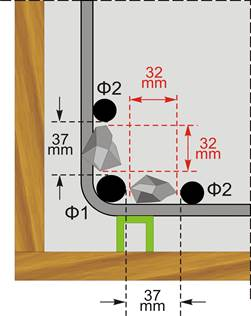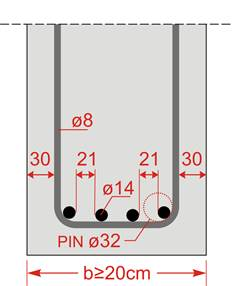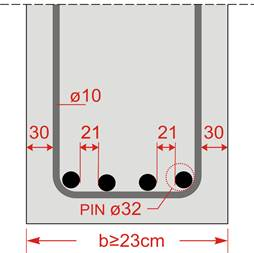# Bar spacingIntroduction >
The structural frame
The construction
The reinforcement I
The reinforcement II
Quantity/Cost estimation
Detailing drawingsIntroduction >
Wind and Seismic Forces >
Structural model and Analysis
Modelling slabs
Slabs
Seismic behavour of frames
Appendix A
Appendix B
Appendix C
Appendix DIntroduction >
Materials
To be continued >

## Minimum bar spacingThe spacing between the reinforcement bars must be adequate in order for the concrete to be casted and compacted successfully and develop sufficient coherence.

The minimum spacing sØ,min between two parallel bars, horizontally or vertically aligned, should be: sØ,min=max{k1×max(Ø1, Ø2), (dg+k2), 20mm}, where dg  is the maximum dimension of the grain of aggregate.

For k1=1.0 and k2 =5mm and as a rule: the maximum grain dimension of ordinary concrete is dg=32mm, while the maximum grain dimension of self-compacting concrete is dg=16mm. Hence:

Ordinary concrete:                               sØ,min =max{max(Ø1, Ø2), 37mm}

self-compacting concrete:                  sØ,min=max{max(Ø1, Ø2), 21mm}

In slabs, generally, there is a large margin since the reinforcement bars are fixed with relatively large spacing between each other.

In earthquake resistant columns, the columns’ dimensions are generally large and the reinforcement bars can be easily fixed in the column’s perimeter.

However, in beams there are usually space constrains that require a careful selection of the beam’s width and, what is more, an accurate placement of reinforcement bars in the node areas.

## Examples